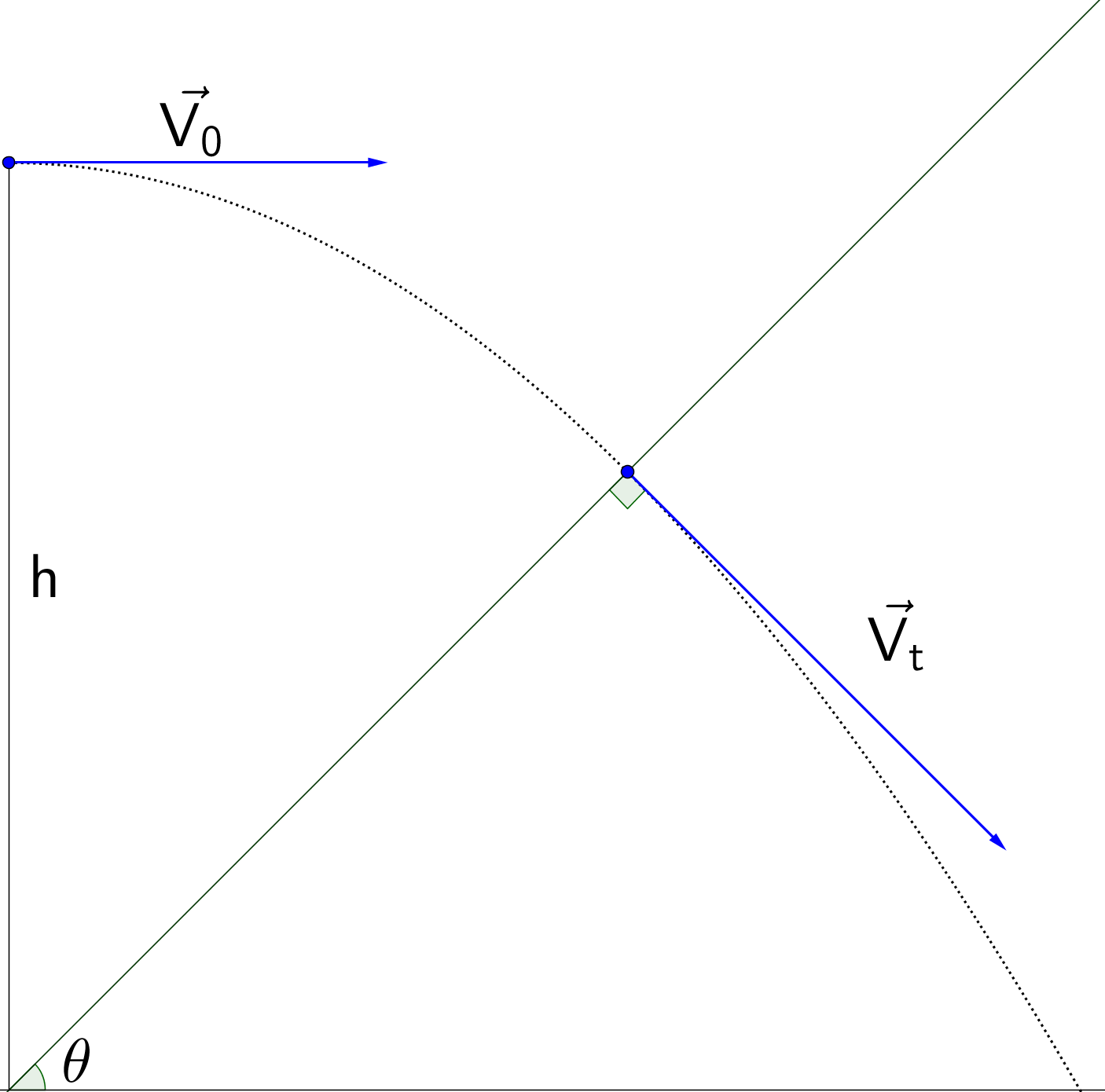Horizontal ShotA particle is thrown horizontally from a height $h$ with initial velocity $V_0$. It hits a plane inclined with an angle $\theta$ with respect to the horizontal. What is the value of $V_0$ such that the particle hits the plane perpendicularly?

×